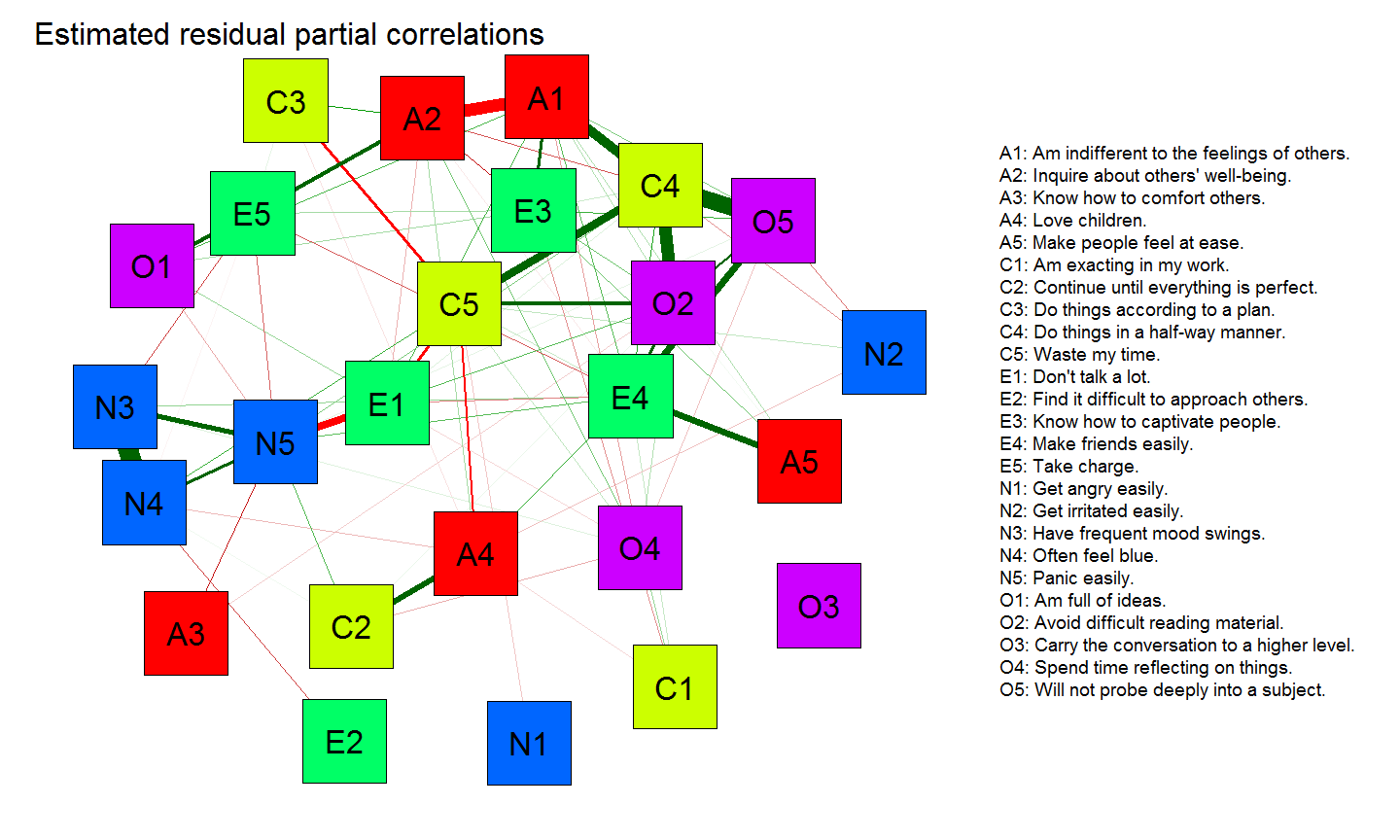## Statistical Modeling of Normal Data

Assume $$\pmb{y}$$, the response on $$P$$ measured items, is centered multivariate Gaussian distributed with variance-covariance matrix $$\pmb{\Sigma}$$:

$\pmb{y} \sim N_P\left( \pmb{0}, \pmb{\Sigma} \right)$

• The goal is to find some model for $$\pmb{\Sigma}$$ with positive degrees of freedom in which $$\pmb{\Sigma}$$ closely resembles the observed variance-covariance matrix
• The number of parameters should be less than $$P(P+1)/2$$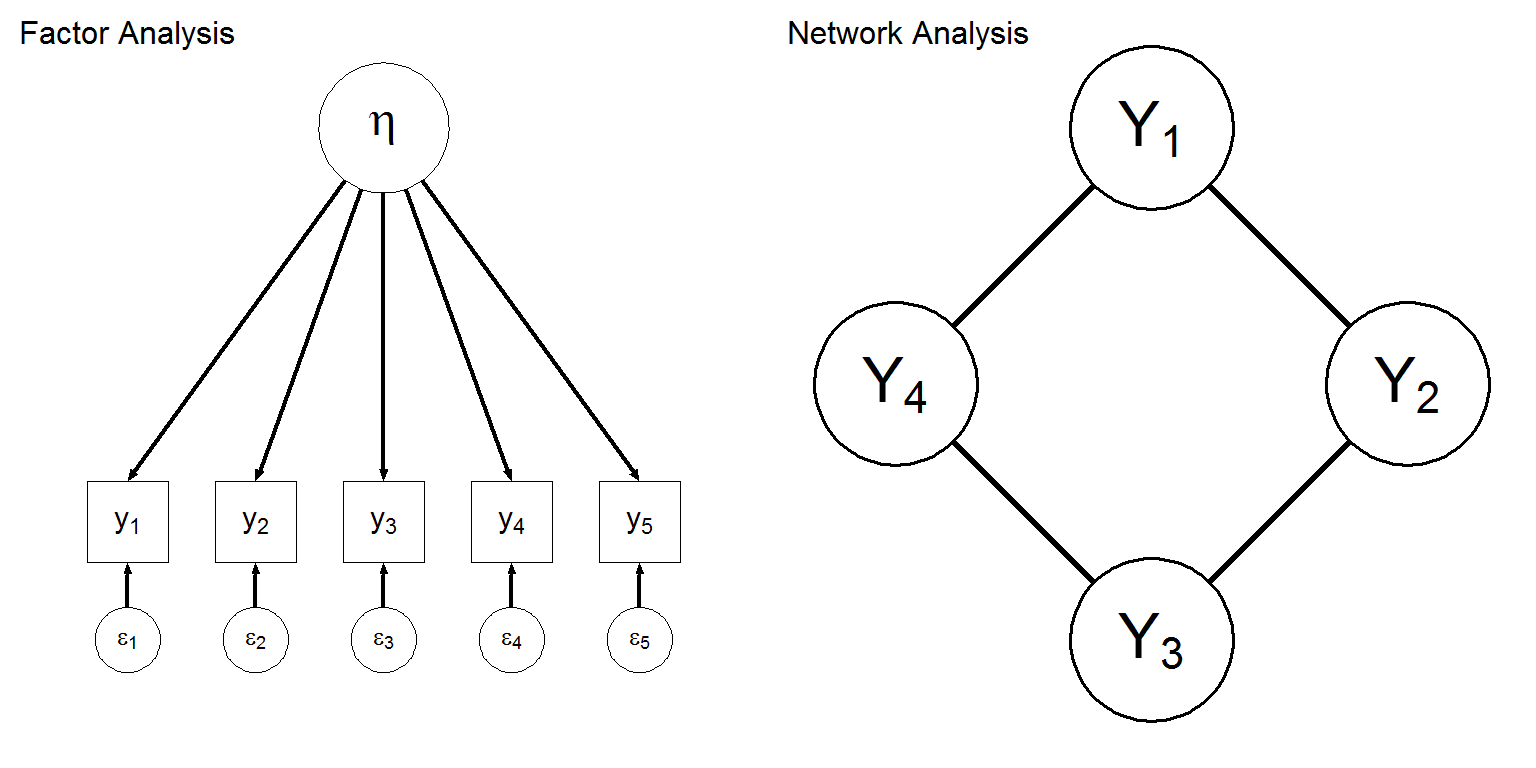## Factor Analysis

$\pmb{\Sigma} = \pmb{\Lambda} \pmb{\Psi} \pmb{\Lambda}^{\top} + \pmb{\Theta}$ With: \begin{aligned} \pmb{\Psi} &= \mathrm{Var}\left( \pmb{\eta} \right) \\ \pmb{\Theta} &= \mathrm{Var}\left( \pmb{\varepsilon} \right) \end{aligned}

• $$\Lambda$$ is a $$P \times M$$ matix containing factor loadings
• Like $$\pmb{\Sigma}$$, $$\pmb{\Theta}$$ is a $$P \times P$$ symmetrical matrix
• To keep the degrees of freedom positive, $$\pmb{\Theta}$$ must mostly contain zeroes
• Local independence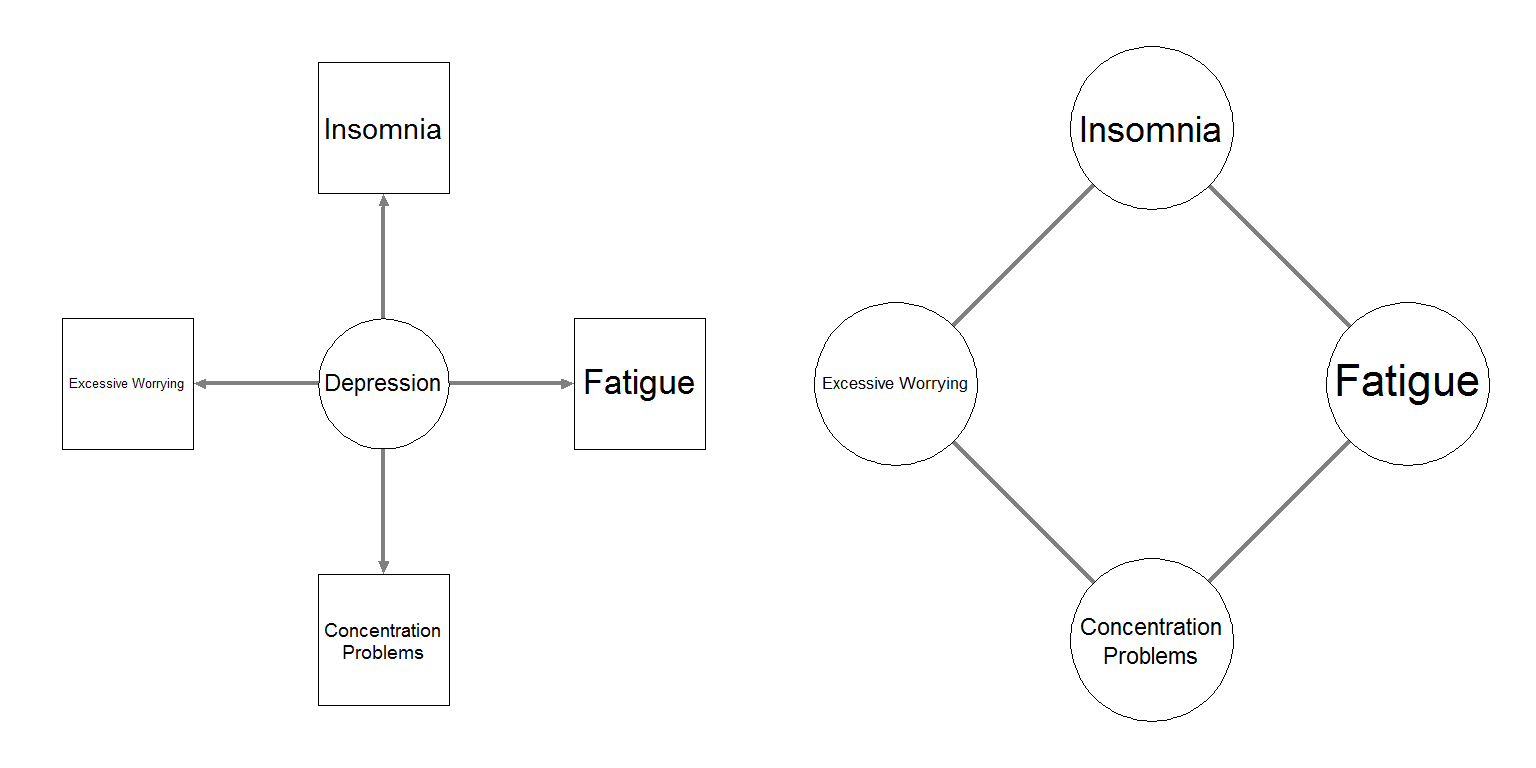• Local independence is not plausible; psychological variables interact with each other
• Allowing these interactions, do we still need latent variables to explain correlatd responses?

## Markov Random Fields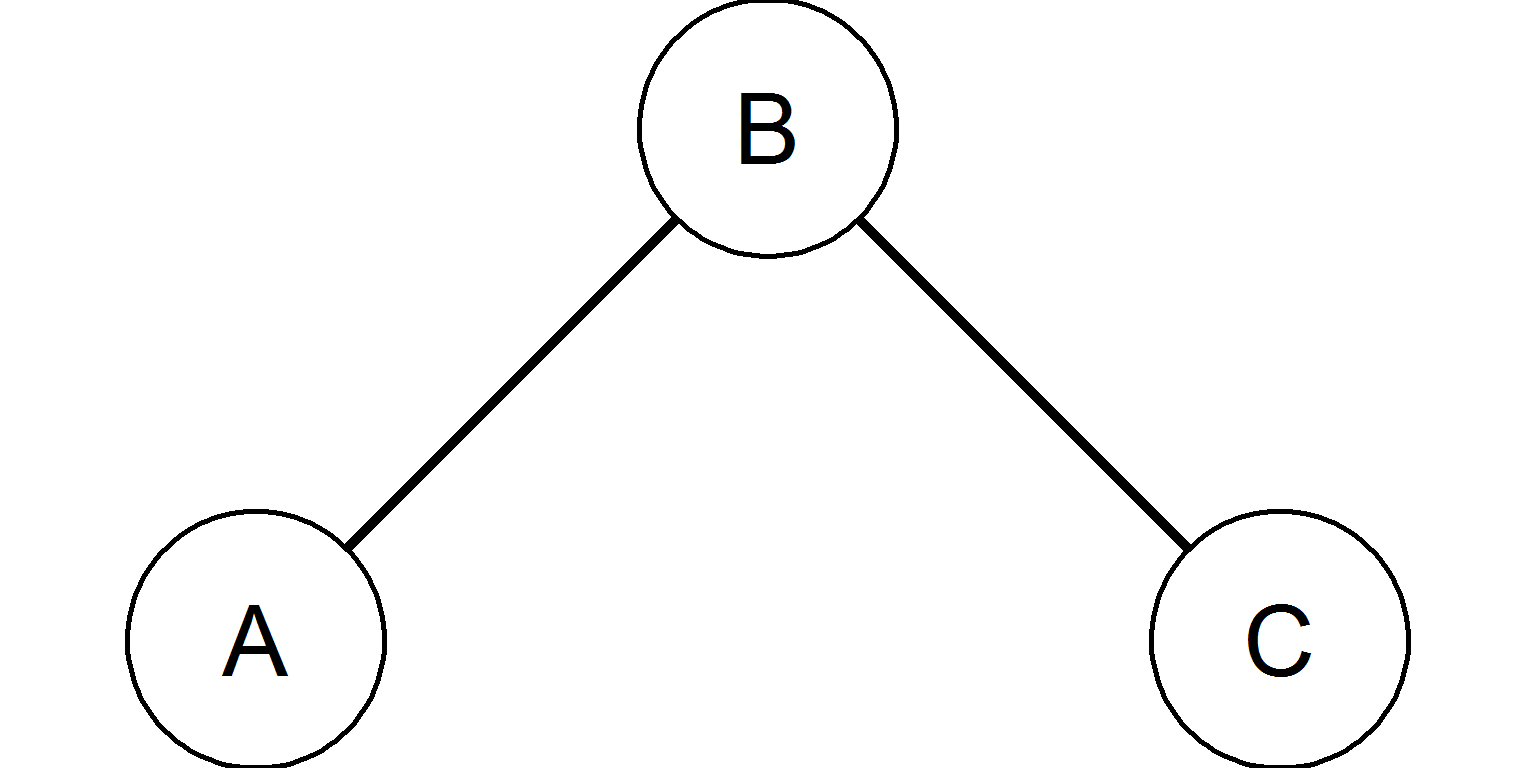• $$A \!\perp\!\!\!\perp C \mid B$$
• Binary data: Ising model
• Gaussian data: Gaussian Graphical Model

## Gaussian Graphical Model

In network analysis, multivariate Gaussian data is modeled with the Gaussian Graphical Model (GGM): $\pmb{\Sigma} = \pmb{\Delta} \left( \pmb{I} - \pmb{\Omega} \right)^{-1}\pmb{\Delta}$

• $$\pmb{\Delta}$$ is a diagonal scaling matrix
• $$\pmb{\Omega}$$ is a $$P \times P$$ symmetrical matrix with $$0$$ on the diagonal and partial correlation coefficients on offdiagonal elements
• $$\omega_{ij} = \omega_{ji} = \mathrm{Cor}\left( Y_i, Y_j \mid \pmb{Y}^{-(i,j)} \right)$$
• Encodes a network; there is no edge between node $$Y_i$$ and $$Y_j$$ if $$\omega_{ij}=0$$
• A GGM is saturated if all offdiagonal elements in $$\pmb{\Omega}$$ are non-zero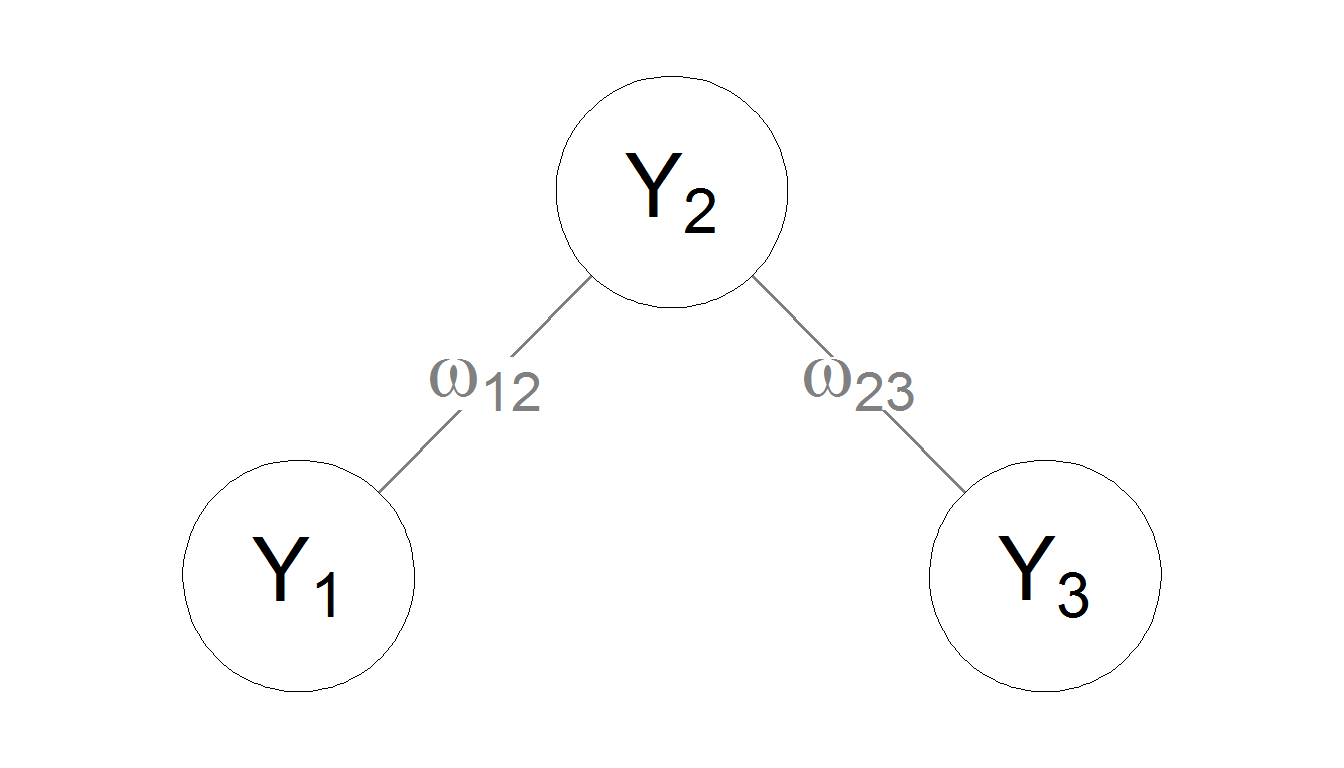$\boldsymbol{\Omega} = \begin{bmatrix} 0 & \omega_{12} & 0\\ \omega_{12} & 0 & \omega_{23}\\ 0 & \omega_{23} & 0\\ \end{bmatrix}$

Sparse configurations of $$\pmb{\Omega}$$ can often lead to dense configurations of $$\pmb{\Sigma}$$

$\boldsymbol{\Omega} = \begin{bmatrix} 0 & 0.5 & 0\\ 0.5 & 0 & 0.5\\ 0 & 0.5 & 0\\ \end{bmatrix}, \pmb{\Delta} = \begin{bmatrix} 1 & 0 & 0\\ 0 & 1 & 0 \\ 0 & 0 & 1 \end{bmatrix}$

Results in:

$\boldsymbol{\Sigma} = \begin{bmatrix} 1.5 & 1 & 0.5 \\ 1 & 2 & 1\\ 0.5 & 1 & 1.5\\ \end{bmatrix}$

• If all nodes are connected, $$\pmb{\Sigma}$$ will be dense

## Critical Assumption: No Latent Variables

Suppose the data is in fact generated by a latent variable model, then the estimated GGM becomes (mail me for proof):

$\pmb{\Omega} = \pmb{I} - \pmb{\Delta} \left( \pmb{\Theta}^{-1} - \pmb{\Theta}^{-1}\pmb{\Lambda} \left(\pmb{\Psi}^{-1} + \pmb{\Lambda}^\top\pmb{\Theta}^{-1}\pmb{\Lambda}\right)^{-1} \pmb{\Lambda}^\top\pmb{\Theta}^{-1} \right) \pmb{\Delta}$

• If a latent variable model did underlie the data, the correct GGM should be dense and thus saturated!

## Problems

• Factor analysis
• Structural violations of the assumption of local independence leads to (near) saturated models
• Network analysis
• Strong violations of the assumption of no latent variables leads to (near) saturated models
• We need a modeling framework that can encompass both!

## Residual Interaction Modeling

The residual variance-covariance matrix in the factor analysis model, $\pmb{\Sigma} = \pmb{\Lambda} \pmb{\Psi} \pmb{\Lambda}^{\top} + \pmb{\Theta},$ can further be modeled as a GGM:

\begin{aligned} \pmb{\Theta} &= \pmb{\Delta}_{\pmb{\Theta}} \left( \pmb{I} - \pmb{\Omega}_{\pmb{\Theta}} \right)^{-1} \pmb{\Delta}_{\pmb{\Theta}} , \end{aligned}

• Because the GGM is a model for pairwise interactions, we call this Residual Interaction Modeling (RIM)
• Since a sparse GGM can lead to a dense variance-covariance matrix, a sparse $$\pmb{\Omega}_{\pmb{\Theta}}$$ can lead to a dense $$\pmb{\Theta}$$
• All residuals can be correlated without being a saturated model

## Residual Interaction Modeling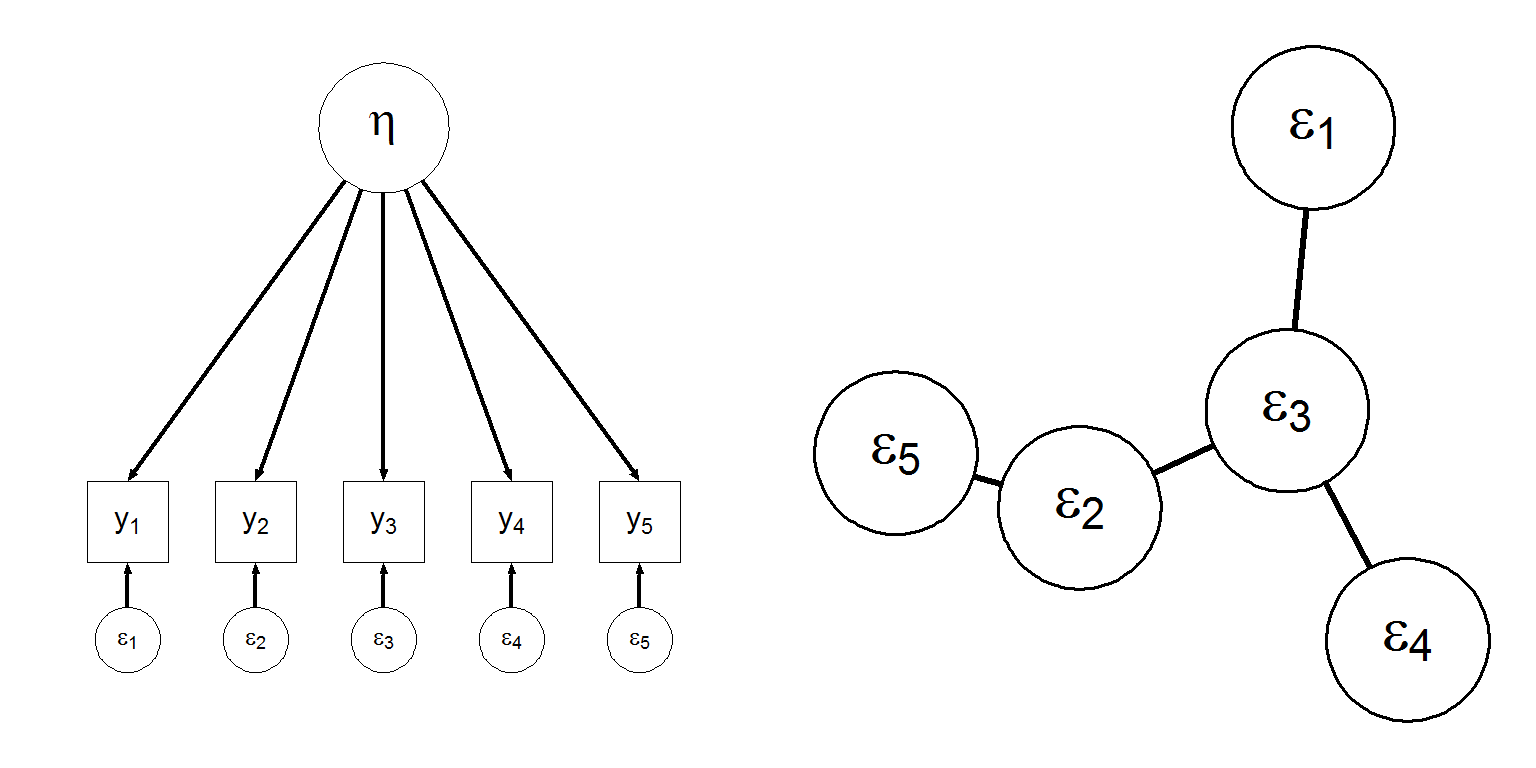## Confirmatory RIM

• Confirmatory estimation of the RIM model (as well as SEM) has been implemented in the "rim" package
• The rim package supports:
• Fit indices
• Model comparison
• Exploratory model search
• rim can also be used for confirmatory estimation of network structures!

## Exploratory RIM

Using a joint vector of observed and latent variables, $$\pmb{u}^{\top} = \begin{bmatrix} \pmb{y}^{\top} & \pmb{\eta}^{\top} \end{bmatrix}$$, we can obtain (mail me for proof): $\mathrm{Var}^{-1}\left( \pmb{u} \right) = \begin{bmatrix} \pmb{\Theta}^{-1} & -\pmb{\Theta}^{-1}\pmb{\Lambda} \\ -\pmb{\Lambda}^\top\pmb{\Theta}^{-1} & \pmb{\Psi}^{-1} + \pmb{\Lambda}^\top\pmb{\Theta}^{-1}\pmb{\Lambda} \end{bmatrix}$

• Encodes a GGM with observed and latent nodes
• Sparse connections assumed between observed nodes
• Estimation of such a model, assuming sparse connections between observed nodes, has been worked out by Chandrasekaran, Parrilo, and Willsky (2010)

## lvglasso

• In a series of paper discussing the work of Chandrasekaran, Parrilo, and Willsky, Yuan described a combination of the glasso algorithm and the EM-algorithm to similarly estimate this model
• This algorithm was called the lvglasso
• Uses the glasso package in R, but was not yet implemented itself in R
• After applying lvglasso, a sparse $$\pmb{\Omega}_{\pmb{\Theta}}$$ and dense $$\pmb{\Lambda}$$ can be obtained
• Combined exploratory factor and network analysis!
• lvglasso has been implemented in the "rim" package (https://github.com/SachaEpskamp/rim)

## Emperical example: personality

I will analyze the BFI dataset from the pych package:

# Load BFI data:
library("psych")
data(bfi)
bfi <- bfi[,1:25]

# Correlation Matrix:
library("qgraph")
bfiCors <- cor_auto(bfi)

# Groups and names objects:
Names <- scan("http://sachaepskamp.com/files/BFIitems.txt",
what = "character", sep = "\n")
Groups <- rep(c('A','C','E','N','O'),each=5)

## Agreeableness

Am indifferent to the feelings of others.

Know how to comfort others.

Love children.

Make people feel at ease.

## Conscientiousness

Am exacting in my work.

Continue until everything is perfect.

Do things according to a plan.

Do things in a half-way manner.

Waste my time.

## Extraversion

Don't talk a lot.

Find it difficult to approach others.

Know how to captivate people.

Make friends easily.

Take charge.

## Neurotocism

Get angry easily.

Get irritated easily.

Have frequent mood swings.

Often feel blue.

Panic easily.

## Openess to Experience

Am full of ideas.

Carry the conversation to a higher level.

Spend time reflecting on things.

Will not probe deeply into a subject.

library("devtools")
install_github("sachaepskamp/rim")
library("rim")
Res <- EBIClvglasso(bfiCors, nrow(bfi), 5)
plot(Res, "network")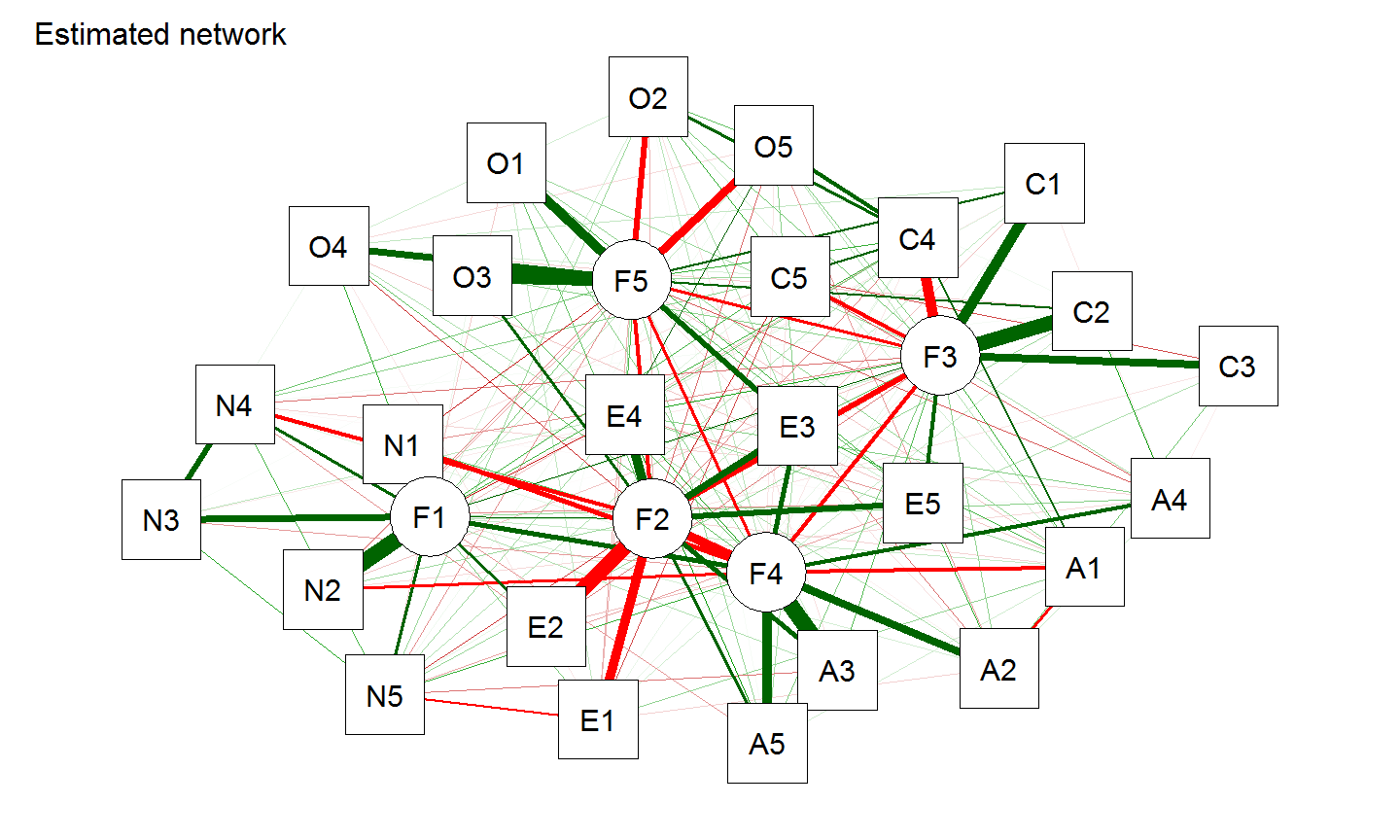plot(Res, "loadings", rotation = promax)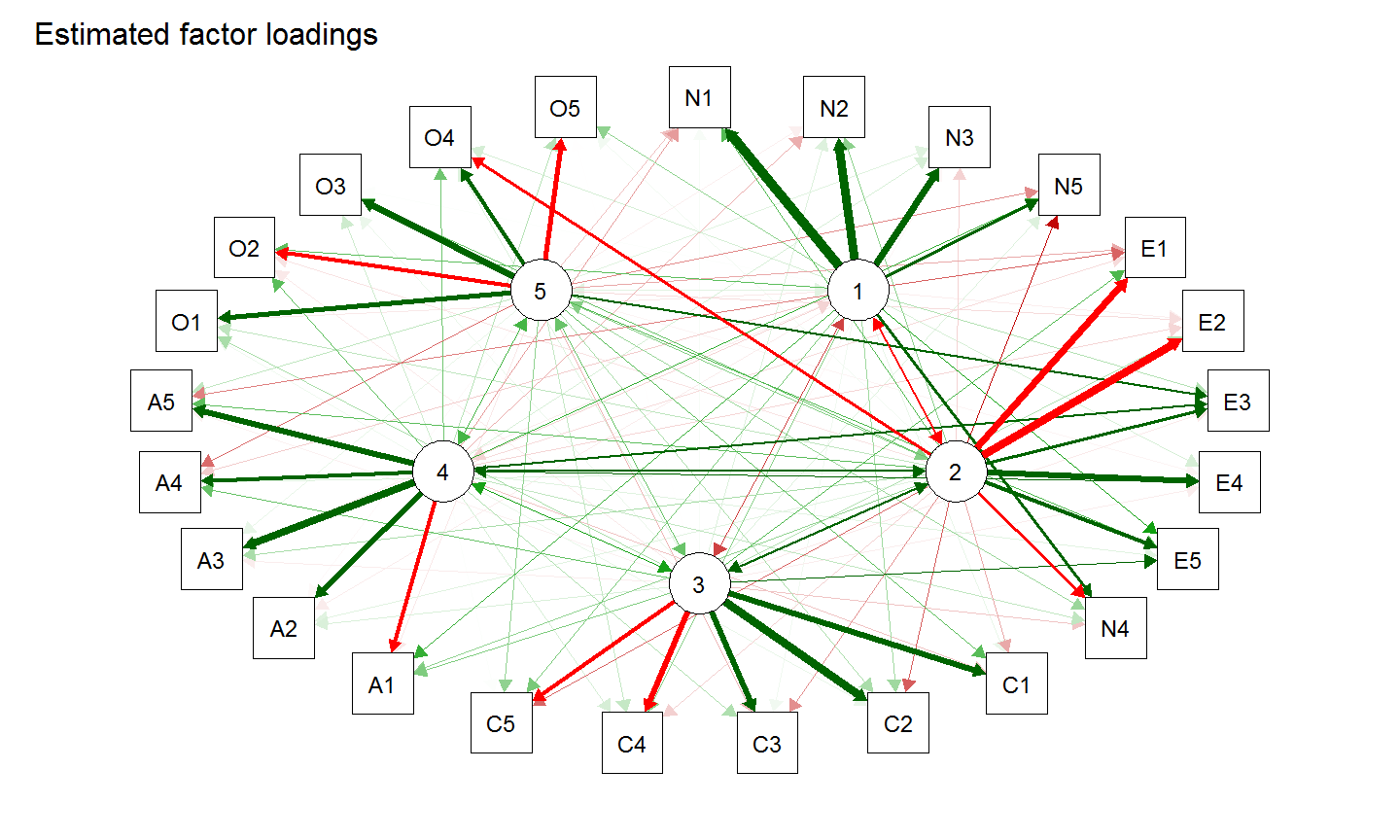plot(Res, "residpcors", nodeNames = Names,
groups = Groups, legend.cex =0.3)